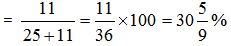Courses

# Quant Mock Test - 13

## 20 Questions MCQ Test Mock Test Series for CLAT 2020 | Quant Mock Test - 13

Description
This mock test of Quant Mock Test - 13 for CLAT helps you for every CLAT entrance exam. This contains 20 Multiple Choice Questions for CLAT Quant Mock Test - 13 (mcq) to study with solutions a complete question bank. The solved questions answers in this Quant Mock Test - 13 quiz give you a good mix of easy questions and tough questions. CLAT students definitely take this Quant Mock Test - 13 exercise for a better result in the exam. You can find other Quant Mock Test - 13 extra questions, long questions & short questions for CLAT on EduRev as well by searching above.
QUESTION: 1

### If both the radius and height of a right circular cone are increased by 20%, its volume will be increased by

Solution: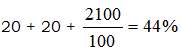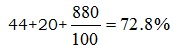QUESTION: 2

### 3 years ago, the average age of family of 5 members was 17 years. A baby having been born, the average age of the family is the same today. The present age of the baby is:

Solution:

3 yr ago, age Sum of Five Member = 85
Therefore present total of five member = 85 + 15 = 100 yr.
After Baby Birth average remain same = 17
∴ 6 Members
Total = 17 x 6 = 102
∴ Baby age = 2 yr.

QUESTION: 3

###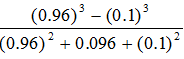is simplified to:

Solution:

(a – b)
= 0.96 – 0.1
= 0.86.

QUESTION: 4

The square root of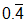is

Solution: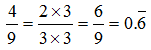QUESTION: 5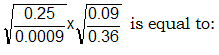Solution: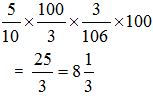QUESTION: 6

The ratio of ages of two students is 3 : 2. One is older to the other by 5 years. What is the age of the younger student?

Solution:
QUESTION: 7

The HCF of two numbers is 23 and the other two factors of their LCM are 13 and 14. The larger of the two numbers is:

Solution:

Larger Number = 23 x 14
= 322.

QUESTION: 8

The next number in the sequence 2, 8, 18, 32, 50, …… is:

Solution: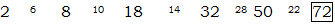QUESTION: 9

Out of 450 students of a school 325 play football, 175 play cricket and 50 neither play football nor cricket, How many students play both football and cricket?

Solution:

The correct answer is B as by drawing vein diagram 100 is the correct answer.

QUESTION: 10

The length of a rectangle is decreased by 10% and its breadth is increased by 10%. By what per cent is its area changed?

Solution: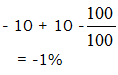QUESTION: 11

A man can row at 5 kmph. in still water. If the velocity of current is 1 kmph and it takes him 1 hour to row to a place and come back, how far is the place?

Solution:

u + v = 6
u – v = 4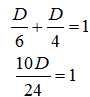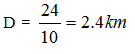QUESTION: 12

The average weight of A, B, C is 45 kg. If the average weight of A and B be 40 kg and that of B and C be 43 kg, then the weight of B is

Solution: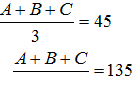80 + C = 135
B = 55
A + B = 80/ B + C = 86
55 + C = 86
C = 31.

QUESTION: 13

A man can do a piece of work in 5 days, but with the help of his son, he can do it in 3 days. In what time can the son do it alone?

Solution:

15 chairs
A = 3 ch
A + B = 5 ch
B = 2 ch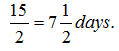QUESTION: 14

Some articles were bought at 6 for Rs. 5, and sold at 5 for Rs. 6. Gain per cent is:

Solution:

C.P = Rs 25
S.P = Rs 36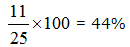QUESTION: 15

Rs. 750 are divided among A, B and C in such a manner that A : B = 5 : 2 and B : C = 7 : 13. What is A’s share?

Solution:

5 : 2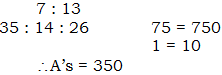QUESTION: 16

A truck covers a distance of 550 metres in 1 minute whereas a bus covers a distance of 33 kms in 45 minutes. The ratio of their speeds is:

Solution: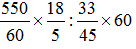3 : 4

QUESTION: 17

If the ratio of areas of two squares is 225:256, then the ratio of their perimeters is:

Solution: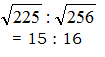QUESTION: 18

If 30% of A = 0.25 of B = 1/5 of C, then A : B : C is equal to:

Solution:

0.30 A = 0.25B = 0.2C
So ratio = 10 : 12 : 15

QUESTION: 19

The percentage increase in the area of a rectangle, if each of its sides is increased by 20% is:

Solution: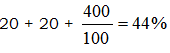QUESTION: 20

A number is increased by 20% and then again by 20%. By what per cent should the increased number be reduced so as to get back the original number?

Solution: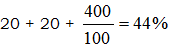Increase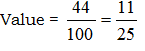Value to be decreased